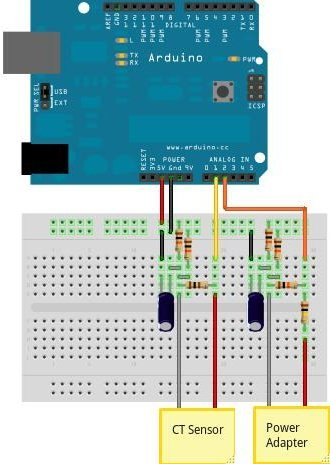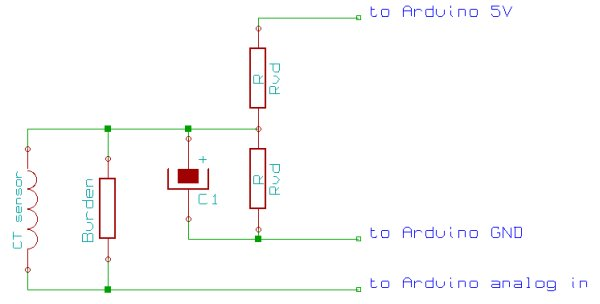# How to build an Arduino energy monitor – measuring mains voltage and current arduino

Including voltage measurement via AC-AC voltage adapter and current measurement via a CT sensor.

This guide details how to build a simple electricity energy monitor on that can be used to measure how much electrical energy you use in your home. It measures voltage with an AC to AC power adapter and current with a clip on CT sensor, making the setup quite safe as no high voltage work is needed.The energy monitor can calculate real power, apparent power, power factor, rms voltage, rms current. All the calculations are done in the digital domain on an Arduino.

### Step One – Gather Components

You will need:

1x Arduino

Voltage sensing electronics:

1x 100kOhm resistor for step down voltage divider.

1x 10kOhm resistor for step down voltage divider.

2x 470kOhm (for voltage divider, any matching value resistor pair down to 10K)

1x 10uF capacitor

Current sensing electronics

1x CT sensor SCT-013-000

1x Burden resistor 18 Ohms if supply voltage is 3.3V or 33 Ohms if supply voltage is 5V.

2x 470kOhm (for voltage divider, any matching value resistor pair down to 10K)

1x 10uF capacitor

Other

1x A breadboard and some single core wire.

Oomlout do a good arduino + breadboard bundle here £29

### Step Two – Assemble the electronics

The electronics consist of the sensors (which produce signals proportional to the mains voltage and current) and the sensor electronics that convert these signals into a form the Arduino is happy with.

For a circuit diagram and detailed discussion of sensors and electronics see:

CT Senors – Introduction

CT Sensors – Interfacing with an Arduino

Measuring AC Voltage with an AC to AC power adapter

Assemble the components as in the diagram above.

### Step Three – Upload the Arduino Sketch

The Arduino sketch is the piece of software that runs on the Arduino. The Arduino converts the raw data from its analog input into a nice useful values and then outputs them to serial.

a) Download EmonLib from github and place in your arduino libraries folder.b) Upload the voltage and current example:

```#include "EmonLib.h"             // Include Emon Library
EnergyMonitor emon1;             // Create an instance

void setup()
{
Serial.begin(9600);

emon1.voltage(2, 234.26, 1.7);  // Voltage: input pin, calibration, phase_shift
emon1.current(1, 111.1);       // Current: input pin, calibration.
}

void loop()
{
emon1.calcVI(20,2000);         // Calculate all. No.of wavelengths, time-out
emon1.serialprint();           // Print out all variables
}```

c) Open the arduino serial window

You should now see a stream of values. These are from left to right: real power, apparent power, rms voltage, rms current and power factor.

#### This Post / Project can also be found using search terms:

• Max471 arduino voltage and current measure